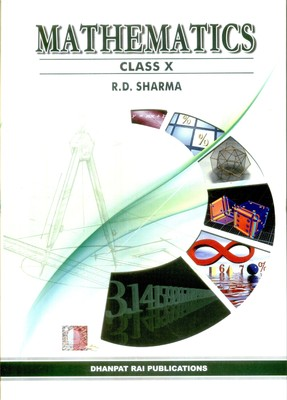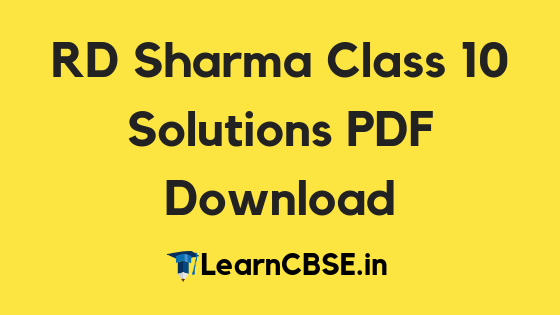## RD Sharma Class 10 Solutions

RD Sharma Class 10 Textbook 2019 is based on the latest syllabus prescribed as per the CCE guidelines by CBSE.  RD Sharma Class 10 pdf Maths book is designed for students who come under the Central Board of Secondary Education (CBSE). RD Sharma solutions were designed in accordance with NCERT guidelines. Free RD Sharma Class 10 Solutions solved by the Maths experts of LearnCBSE.in following up the (NCERT) CBSE guidelines. Students can easily score more marks in Class 10 Maths and Class 10 Science.• Detailed Step by Step explanation for each exercise.
• Complete answers for each question in RD Sharma Class 10.
• 1466 Solved Questions from RD Sharma Class 10th Mathematics.

### RD Sharma Class 10 Solutions Polynomials

• Polynomial – Let x be a variable, n be a positive integer and a1, a2, a3 ……… an be constants (real numbers), then f (x) = an xn + an-1 xn-1 + an-2 xn-2 + ……… + a1x+ a0 is called a polynomial in variable x.
• an xn, an-1 xn-1, an-2 xn-2 ………  a1x, a0 are known as the terms of the polynomial and an, an-1 …….a1, a0 are their co-efficients.
• Degree of Polynomial – The exponent of the highest degree term in a polynomial is known as its degree.
• Constant Polynomial – A polynomial of degree zero (0) is called a constant polynomial.
• Linear Polynomial – A polynomial of degree 1 is called the linear polynomial.
• A linear polynomial may be monomial or a binomial having one term or two terms respectively e.g., ax or ax + b.
• Zero of a Polynomial: For any polynomial p(x), if p(k) = 0, k is called a zero of polynomial p(x).
• Number of zeroes/roots of a polynomial p(x) is always equal to or less than the degree of polynomial p(x).
• If a polynomial p(x) is divided by (x — a), then remainder will be p(a) = r.
• If a polynomial p(x) is divisible by (x — a), then p(a) = O.

### RD Sharma Class 10 Solutions Chapter 3

• Linear equation: An equation of the form ax + by + c = O, where a, b, c all are real numbers and a ≠ 0, b ≠ 0, is known as a linear equation in two variables x and y. Its graph is always a straight line.
• System of Simultaneous Linear Equations: A pair of linear equations in two variables a1x + b1y + c1= 0 and a2x + b2y + c2= 0 is said to form a system of simultaneous linear equations, where a1, a2, b1, b2, c1, c2 are real numbers.
• A pair of values of x and y which satisfy each of the equations is called a solution or root of the system.
• If the system has at least one solution, it is called consistent and if the system has no solution, it is called inconsistent.
• A pair of linear equations in two variables can be solved by the :
Graphical method— To solve a pair of linear equations in two variables by Graphical method, we first draw the lines represented by them.
• If the pair of lines intersect at a point, then we say that the pair is consistent and the coordinates of the point provide us the unique solution.
• If the pair of lines are parallel, then the pair has no solution and is called inconsistent pair of equations.
• If the pair of lines are coincident, then it has infinitely many solutions-each point on the line being of solution. In this case, we say that the pair of lines equations is consistent with infinitely many solutions.
• Algebraic method—To solve a pair of linear equations in two variables algebraically, we have (i) Substitution method (ii) Elimination method (iii) Cross-multiplication method.

### RD Sharma Class 10 Solutions Trigonometric Identities

• Trigonometrical Identity: An equation, involving trigonometric ratios of ane angle and which is true for all values of the angle, is called a trigonometrical identity.
• While solving the trigonometric identities, keep in mind the necessary algebraic identities and learn using them properly. Practise thoroughly how to use the algebraic expressions in solving the trigonometric identities.

### RD Sharma Class 10 Solutions Trigonometric Ratios

• Trigometric Ratios: In the right angled triangle AMP, Base = AM = x, Perpendicular = PM = y, and Hypotenuse = AP = r.
• It should be noted that sin θ is an abbreviation for “sine of angle θ”, it is not the product of sin and θ. Similar is the case for other trigonometric ratios.
• The trigonometric ratios are defined for an acute angle θ.
• The trigonometric ratios depend only on the value of angle θ.
• Sometimes due to common mistake, the wrong usage of the trigonometric ratios is done in solving/proving trigonometric identities. Try to memorize all the trigonometric ratios by your own way to overcome this situation.

### RD Sharma Class 10 Solutions Arithmetic Progression

• Sequence: A sequence is an arrangement of terms, which are formed according to some definite patterns. From a definite pattern, we can find the general term (in terms of n).
• Terms: The elements in a sequence are called terms. A sequence is generally written as tn, where tn is nth term and t1, t2, … are first, second,… terms of the sequence.
• Finite Sequence: A sequence containing finite number of terms is called a finite sequence.
• Infinite Sequence: A sequence containing infinite number of terms is called an infinite sequence.
• Arithmetic Progression (AP): Arithmetic progression is a sequence in which each term, except the first term, differs from its preceding term by a fixed number (constant). The constant or fixed number is called the common difference of the arithmetic progression.
• Generally, an arithmetic progression with first term a and common difference d is represented as a, a + d, a + 2d, a + 3d, ….
• To find nth Term of an AP: The nth term of an AP with first term a and common difference d is given by tn = a + (n – 1) d.

Mathematics For Class 10 By R D Sharma Pdf

RD Sharma and NCERT Video Solutions

### FAQs on RD Sharma Class 10 Solutions

Yes, just one click is enough to download RD Sharma Class 10 Solutions PDF from our website @ learnCBSE.in

2. Which is the best resource for scoring better marks in the board exams?

There are plenty of exam resources available in the market & online, but preparing with RD Sharma Book Solutions will help you to the fullest and score better marks in Class 10 and competitive exams.

3. Where can I avail RD Sharma Chapter-wise Solutions for Class 10?

Aspirants are advised to check our website LearnCBSE.in to get all chapters RD Sharma Class 10 Solutions for free.

4. Is solving RD Sharma Class 10 Solutions enough for board exam preparation?